# Class 6 Maths Mensuration Notes

In this page we have Notes for Class 6 Maths mensuration . Hope you like them and do not forget to like , social share and comment at the end of the page.

## Perimeter

Perimeter is the distance covered along the boundary forming a closed figure when you go round the figure once.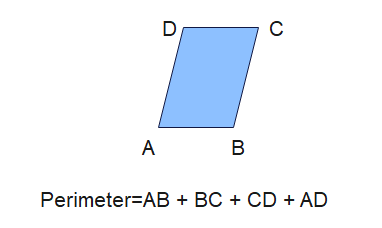## Perimeter Of rectangle

Perimeter Of rectangle is given by the Formula
$P=2 (L + B)$
Where L and B are the length and Breadth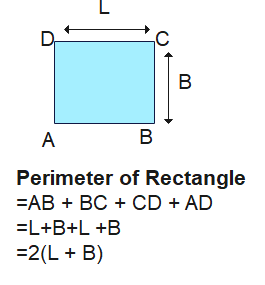## Perimeter Of Regular Shapes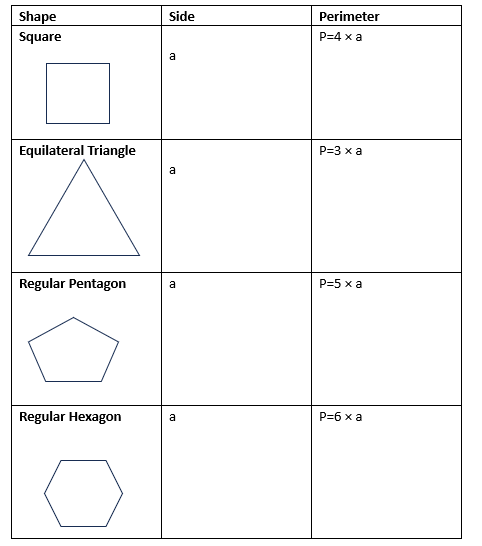## Area

• The amount of surface enclosed by a closed figure is called its area.
• It is measured in number of square of 1 m or 1cm in the enclosed area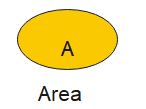## How To estimate Area

We estimate the area using the below rules
• Place the figure on a squared paper or graph paper where every square measures 1 cm × 1 cm.
• Now find out the number of squares using below rules
• The area of one full square is taken as 1 sq unit. If it is a centimetre square sheet, then area of one full square will be 1 sq cm.
• Ignore portions of the area that are less than half a square.
• If more than half of a square is in a region, just count it as one square.
• If exactly half the square is counted, take its area as 1/2 sq cm

## Area of Rectangle

Area Of rectangle is given by the Formula
$A=L\times B$
Where L and B are the length and Breadth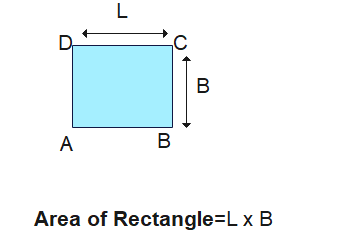## Area of Square

Area Of Square is given by the Formula
$A=4 \times L$
Where L is the side of the square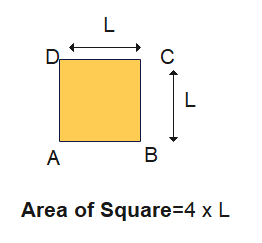## Examples

Example 1: Rectangle Perimeter
A rectangle has a length of 10 cm and a breadth of 6 cm. Find its perimeter.
Solution:
$P = 2(l+b)$
$P = 2(10 + 6)$
$P = 2(16)$
$P = 32$ cm

Example 2: Square Area
A square has a side length of 4 cm. Find its area.
Solution:
$A = s^2$
$A = 4^2$
$A = 16$ sq cm

Example 3: Equilateral Triangle Perimeter
A Equilateral triangle has sides of length 7 cm. Find its perimeter.
Solution:
$P = a+b+c$
$P = 7 + 7 + 7$
$P = 21$ cm

Example 4: Pentagon Perimeter
A Pentagon triangle has sides of length 11 cm. Find its perimeter.
Solution:
$P = 5 \times L$
$P = 55$cm

## Area of Composite shapes

• While calculating the area of composite figures, you can break down the shape into simpler shapes, calculate their individual areas, and then add/subtract them as needed.
Example By splitting the following figures into rectangles, find the area (The measures are given in centimetres)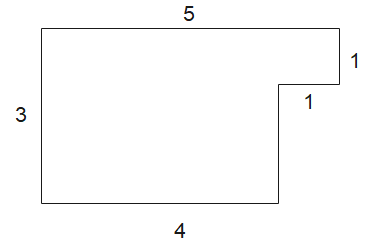Solution:
We can split that in 1 square of 1 cm and 1 rectangle of 3 x 4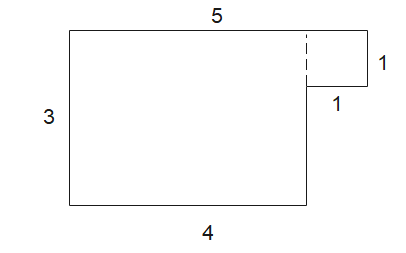So Area= 1 + 12= 12 sq cm### Practice Question

Question 1 What is $\frac {1}{2} + \frac {3}{4}$ ?
A)$\frac {5}{4}$
B)$\frac {1}{4}$
C)$1$
D)$\frac {4}{5}$
Question 2 Pinhole camera produces an ?
A)An erect and small image
B)an Inverted and small image
C)An inverted and enlarged image
D)None of the above